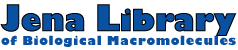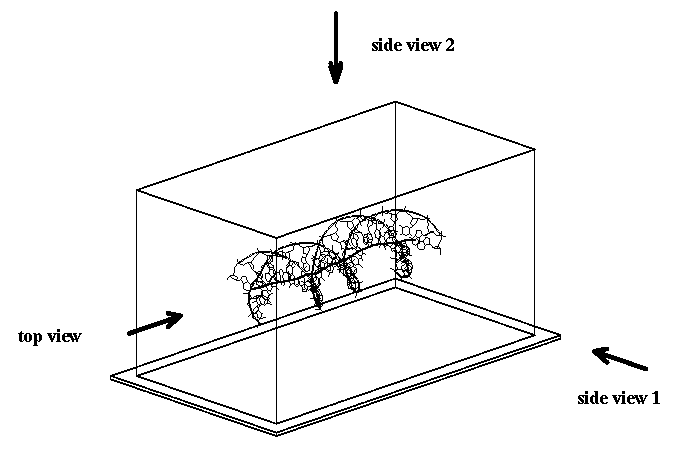Help Orthogonal views

# Orthogonal views

For the visualization of nucleic acid double helix structures we use a presentation where three orthogonal views are shown. These views are called top view, side view 1 and side view 2. If you look along the helical axis this view is called top view. On the other hand, if you view perpendicular to the helical axis these views are called side view 1 and side view 2 (Figure 1).Figure 1.  Definition of side and top views.

The curvilinear axis of the helix is calculated with CURVES. This yields one reference point for each base pair. The moment of inertia is calculated using an equal mass for every point of the helical axis. Then we determine the principle axes of the curvilinear helical axis. These axes are used to orient the double helix. The lowest principle moment of inertia is then assumed to be about the x-axis, the second lowest about the y-axis, and the highest about the z-axis. This means that for an ideal double helix the helical axis is identical to the x-axis of the Cartesian coordinate system. This assignment has some useful implications for the visualization of bending as described in the Bending analysis help page.

 Perl script: help.pl  (22 Mar 1999) Authors: Peter Slickers and Jürgen Sühnel  (slickers@imb-jena.de, jsuehnel@imb-jena.de),  IMB Jena,  Germany